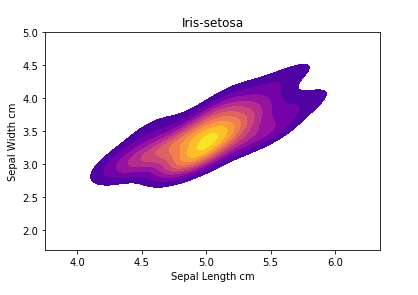﻿ Python Machine learning Scikit-learn: Create a kde plot of sepal_length versus sepal width for setosa species of flower - w3resource# Python Scikit-learn: Create a kde plot of sepal_length versus sepal width for setosa species of flower

## Python Machine learning Iris Visualization: Exercise-14 with Solution

Write a Python program using seaborn to Create a kde (Kernel Density Estimate ) plot of sepal_length versus sepal width for setosa species of flower.

Sample Solution:

Python Code:

``````# Import necessary modules
import pandas as pd
import seaborn as sns
import matplotlib.pyplot as plt
#Drop id column
iris = iris.drop('Id',axis=1)
sub=iris[iris['Species']=='Iris-setosa']
plt.title('Iris-setosa')
plt.xlabel('Sepal Length cm')
plt.ylabel('Sepal Width cm')
plt.show()
```
```

Output:

``````

Python Code Editor:

Have another way to solve this solution? Contribute your code (and comments) through Disqus.

Next: Write a Python program using seaborn to create a kde (Kernel Density Estimate ) plot of petal_length versus petal width for setosa species of flower.

What is the difficulty level of this exercise?

﻿